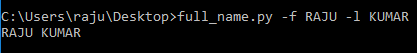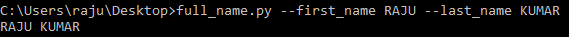GeeksforGeeks App
Open AppBrowser
Continue

# Getopt module in Python

The getopt module is a parser for command-line options based on the convention established by the Unix `getopt()` function. It is in general used for parsing an argument sequence such as sys.argv. In other words, this module helps scripts to parse command-line arguments in sys.argv. It works similar to the C `getopt()` function for parsing command-line parameters.

## Python getopt function

The first function provided by this module is of the same name i.e. `getopt()`. Its primary functionality is to parse command-line options and parameter list. The syntax of the function is as below:

Syntax: getopt.getopt(args, options, [long_options])

Parameters:
args: List of arguments to be passed.
options: String of option letters that the script wants to recognize. Options that require an argument should be followed by a colon (:).
long_options: List of the string with the name of long options. Options that require arguments should be followed by an equal sign (=).

Return Type: Returns value consisting of two elements: the first is a list of (option, value) pairs. The second is the list of program arguments left after the option list was stripped.

Example 1:

 `import` `sys``import` `getopt`` ` ` ` `def` `full_name():``    ``first_name ``=` `None``    ``last_name ``=` `None`` ` `    ``argv ``=` `sys.argv[``1``:]`` ` `    ``try``:``        ``opts, args ``=` `getopt.getopt(argv, ``"f:l:"``)``     ` `    ``except``:``        ``print``(``"Error"``)`` ` `    ``for` `opt, arg ``in` `opts:``        ``if` `opt ``in` `[``'-f'``]:``            ``first_name ``=` `arg``        ``elif` `opt ``in` `[``'-l'``]:``            ``last_name ``=` `arg``     ` ` ` `    ``print``( first_name ``+``" "` `+` `last_name)`` ` `full_name()    `

Output:Here we have created a function `full_name()`, which prints the full name after getting first name and last name from the command line. We have also abbreviated first name as ‘f’ and last name as ‘l’.

Example 2: Now lets look into the case where instead of short-form like ‘f’ or ‘l’, we could use full forms as ‘first_name’ and ‘last_name’.The below code uses full forms to print full name;

 `import` `sys``import` `getopt`` ` `def` `full_name():``    ``first_name ``=` `None``    ``last_name ``=` `None`` ` `    ``argv ``=` `sys.argv[``1``:]`` ` `    ``try``:``        ``opts, args ``=` `getopt.getopt(argv, ``"f:l:"``, ``                                   ``[``"first_name ="``,``                                    ``"last_name ="``])``     ` `    ``except``:``        ``print``(``"Error"``)`` ` `    ``for` `opt, arg ``in` `opts:``        ``if` `opt ``in` `[``'-f'``, ``'--first_name'``]:``            ``first_name ``=` `arg``        ``elif` `opt ``in` `[``'-l'``, ``'--last_name'``]:``            ``last_name ``=` `arg``     ` ` ` `    ``print``( first_name ``+``" "` `+` `last_name)`` ` `full_name()`

Output:Note: The single dash(‘-‘) for short forms and double dash(‘–‘) for long form of the argument in the code should be used.

My Personal Notes arrow_drop_up